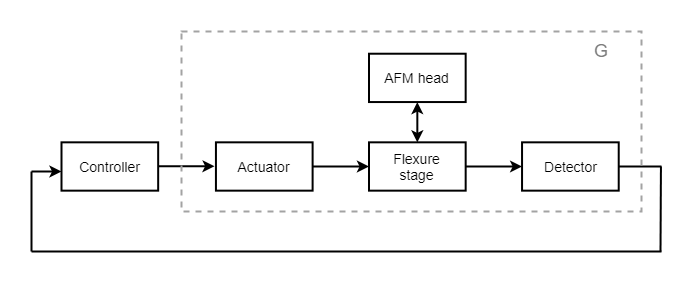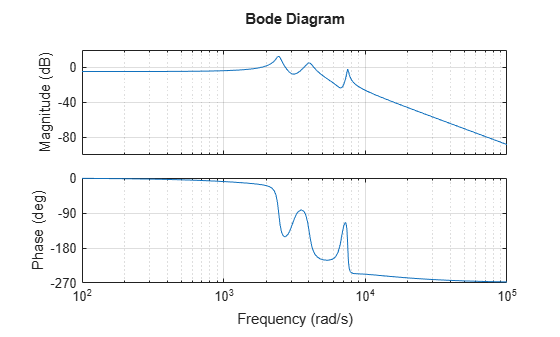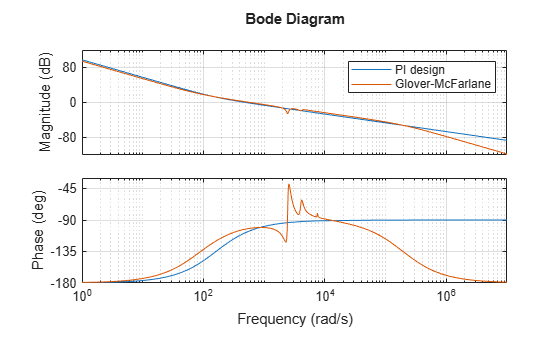# Robust Loop Shaping of Nanopositioning Control System

This example shows how to use the Glover-McFarlane technique to obtain loop-shaping compensators with good stability margins. The example applies the technique to a nanopositioning stage. These devices can achieve very high precision positioning which is important in applications such as atomic force microscopes (AFMs). For more details on this application, see .

### Nanopositioning System

The following illustration shows a feedback diagram of a nanopositioning device. The system consists of piezo-electric actuation, a flexure stage, and a detection system. The flexure stage interacts with the head of the AFM.Load the plant model for the nanopositioning stage. This model is a seventh-order state-space model fitted to frequency response data obtained from the device.

```load npfit A B C D G = ss(A,B,C,D); bode(G), grid```Typical design requirements for the control law include high bandwidth, high resolution, and good robustness. For this example, use:

• Bandwidth of approximately 50 Hz

• Roll-off of -40 dB/decade past 250 Hz

• Gain margin in excess of 1.5 (3.5 dB) and phase margin in excess of 60 degrees

Additionally, when the nanopositioning stage is used for scanning, the reference signal is triangular, and it is important that the stage tracks this signal with minimal error in the midsection of the triangular wave. One way of enforcing this is to add the following design requirement:

• A double integrator in the control loop

### PI Design

First try a PI design. To accommodate the double integrator requirement, multiply the plant by $1/s$. Set the desired bandwidth to 50 Hz. Use `pidtune` to automatically tune the PI controller.

```Integ = tf(1,[1 0]); bw = 50*2*pi; % 50 Hz in rad/s PI = pidtune(G*Integ,'pi',50*2*pi); C = PI*Integ; bopt = bodeoptions; bopt.FreqUnits = 'Hz'; bopt.XLim = [1e0 1e4]; bodeplot(G*C,bopt), grid```This compensator meets the bandwidth requirement and almost meets the roll-off requirement. Use `allmargin` to calculate the stability margins.

`allmargin(G*C)`
```ans = struct with fields: GainMargin: [0 1.1531 13.7832 7.4195 Inf] GMFrequency: [0 2.4405e+03 3.3423e+03 3.7099e+03 Inf] PhaseMargin: 60.0024 PMFrequency: 314.1959 DelayMargin: 0.0033 DMFrequency: 314.1959 Stable: 1 ```

The phase margin is satisfactory, but the smallest gain margin is only 1.15, far below the target of 1.5. You could try adding a lowpass filter to roll off faster beyond the gain crossover frequency, but this would most likely reduce the phase margin.

### Glover-McFarlane Loop Shaping

The Glover-McFarlane technique provides an easy way to tweak the candidate compensator `C` to improve its stability margins. This technique seeks to maximize robustness (as measured by `ncfmargin`) while roughly preserving the loop shape of `G*C`. Use `ncfsyn` to apply this technique to this application. Note that `ncfsyn` assumes positive feedback so you need to flip the sign of the plant `G`.

`[K,~,gam] = ncfsyn(-G,C);`

Check the stability margins with the refined compensator `K`.

`[Gm,Pm] = margin(G*K)`
```Gm = 3.7267 ```
```Pm = 70.7109 ```

The `ncfsyn` compensator increases the gain margin to 3.7 and the phase margin to 70 degrees. Compare the loop shape for this compensator with the loop shape for the PI design.

```bodeplot(G*C,G*K,bopt), grid legend('PI design','Glover-McFarlane')```The Glover-McFarlane compensator attenuates the first resonance responsible for the weak gain margin while boosting the lead effect to preserve and even improve the phase margin. This refined design meets all requirements. Compare the two compensators.

```bodeplot(C,K,bopt), grid legend('PI design','Glover-McFarlane')```The refined compensator has roughly the same gain profile. `ncfsyn` automatically added zeros in the right places to accommodate the plant resonances.

### Compensator Simplification

The `ncfsyn` algorithm produces a compensator of relatively high order compared to the original second-order design.

`order(K)`
```ans = 11 ```

You can use `ncfmr` to reduce this down to something close to the original order. For example, try order 4.

```ord = 4; Kr = ncfmr(K,ord); [Gm,Pm] = margin(G*Kr)```
```Gm = 3.8139 ```
```Pm = 70.6770 ```
```bodeplot(G*K,G*Kr,bopt), grid legend('11th order','4th order')```The reduced-order compensator `Kr` has very similar loop shape and stability margins and is a reasonable candidate for implementation.

### References

1. Salapaka, S., A. Sebastian, J. P. Cleveland, and M. V. Salapaka. “High Bandwidth Nano-Positioner: A Robust Control Approach.” Review of Scientific Instruments 73, no. 9 (September 2002): 3232–41.